Prime Factorization 4
Set 4 (10 Questions)
Determine the prime factors of all numbers through 50 and write the numbers as the product of their prime factors by using exponents to show multiples of a factor.
From Mr. Anker Tests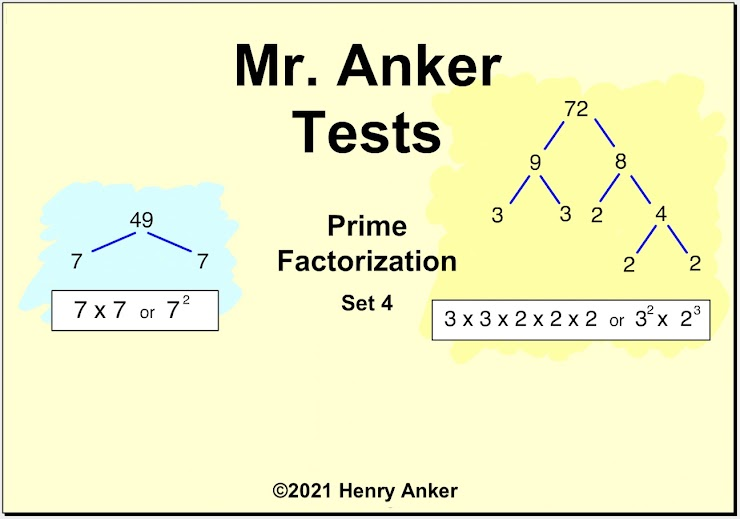Prime Factorization Help Slide 1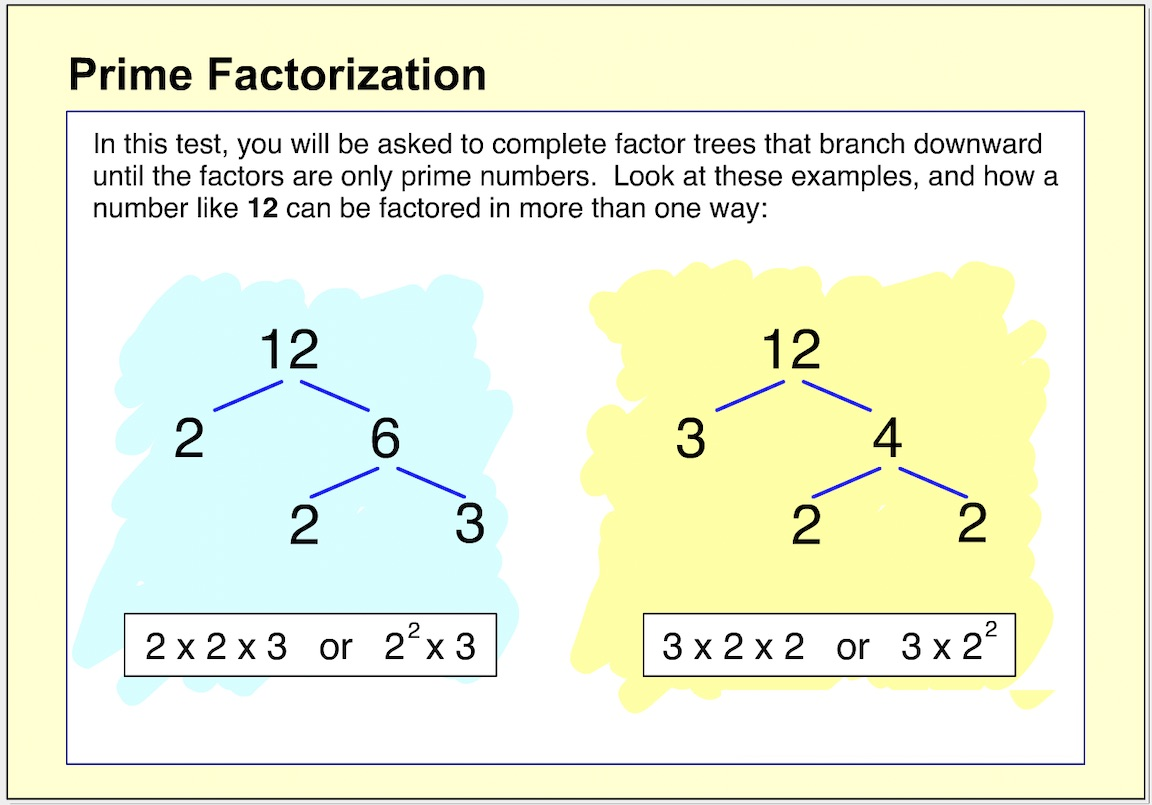Prime Factorization Help Slide 2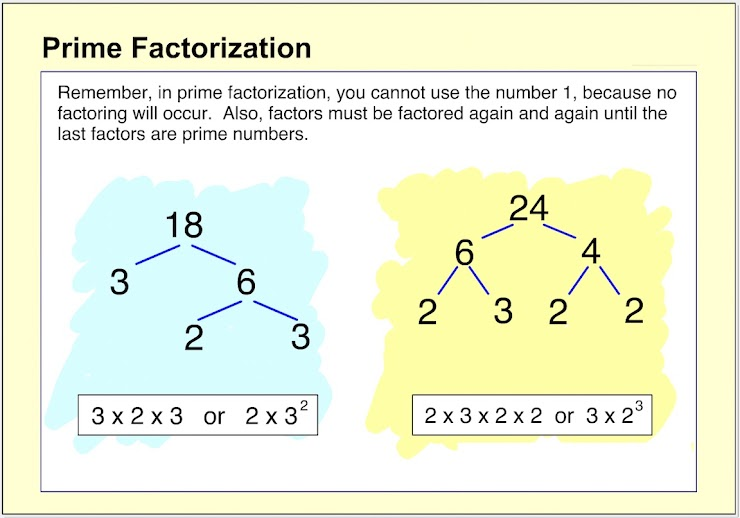Prime Factorization Help Slide 3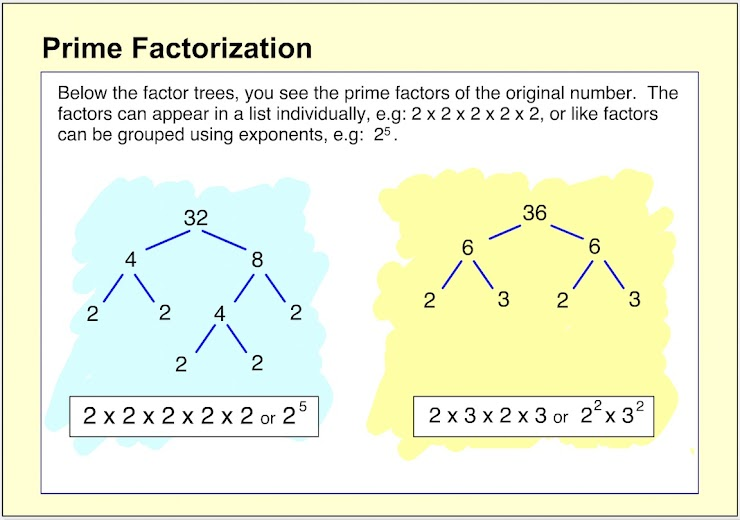Prime Factorization Help Slide 4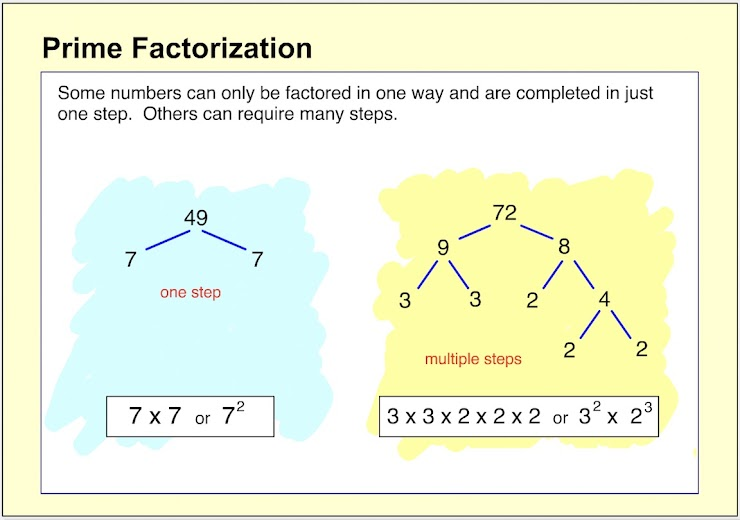Prime Factorization Help Slide 5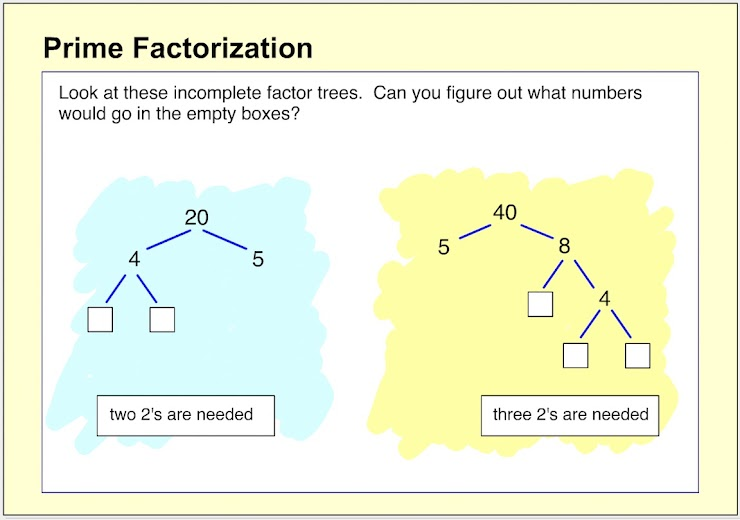1.  On the line below, type in the exponent value to complete the prime factorization. *
1 point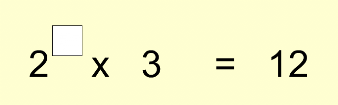2. On the line below, type in the exponent value to complete the prime factorization. *
1 point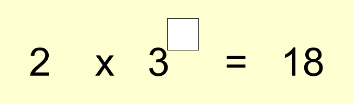3. On the line below, type in the exponent value to complete the prime factorization. *
1 point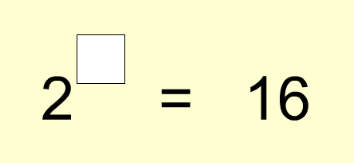4. On the line below, type in the exponent value to complete the prime factorization. *
1 point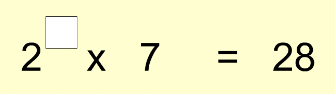5.  What is the prime factorization of 30? *
1 point
6.  What is the prime factorization of 36? *
1 point
7.  What is the prime factorization of 42? *
1 point
8.  What is the prime factorization of 50? *
1 point
9.  What is the prime factorization of 36? *
1 point
10.  What is the prime factorization of 100? *
1 point
Submit
Clear form